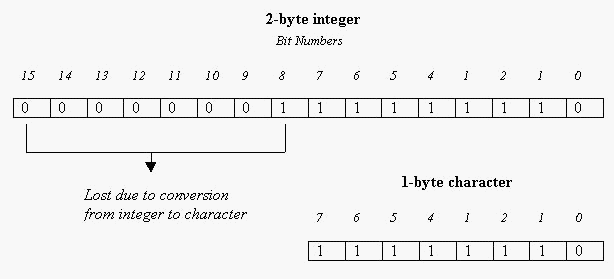C++ Operators - II

The following topics are covered in this section:

2. Assignment Operators

The assignment operator assigns the value on its right-hand side to whatever is present on the left-hand side. The ‘equal to’ symbol ( = ) is the C++ assignment operator.

For example, the statement

x = 2;

assigns the value on the right-hand side of the ‘equal to’ sign to the term on the left (i.e. the value 2 is assigned to the variable x). The term which appears on the left-hand side of the assignment operator (usually a variable) is called the ‘target’.

You will always assign a value to some variable but not to a constant.

2 = x;         //Error

is a wrong statement because you cannot assign the value of x to 2 (2 is an integer constant and you cannot change its value).

The value on the right side of the assignment operator can be an expression as shown below (or a function that returns a value):
x = num1 + num2;
y = 5*6;

Compilers often use the terminology ‘lvalue’ (or Lvalue) and ‘rvalue’ especially in error messages. The ‘lvalue’ refers to the operand on the left-hand side of the assignment operator while the ‘rvalue’ refers to the operand on the right-hand side of the assignment operator. The lvalue can be a variable or a pointer but it should not be a constant. The rvalue can be a constant, variable, an expression or a function call.

Beware: Lvalue can be used as rvalue (i.e. on the right-hand side of an assignment) but rvalue cannot be used as Lvalue.

C++ permits multiple assignments (i.e. more than one variable can be assigned a value using one statement). For example let x, y and z be integers, then

x = y = z = 3;

is an example of multiple assignment. The process of assignment will be from right to left; 3 is assigned to ‘z’ and the value of ‘z’ (which is now 3) is assigned to ‘y’ and y’s value is assigned to ‘z’. The above multiple assignment is equivalent to the following set of statements:

z = 3;
y = z;
x = y;

and all the three variables x,y and z are assigned the value of 3.

Is this valid?

x = y+1 = 3;

This sort of a statement is not valid in C++. You cannot use arithmetic operators in between multiple assignments (the compiler will not know how to solve this statement).

Type Conversion

Each data type has a different range because of the different sizes that they occupy. A character is allotted 1 byte while an integer might be allotted 2/4 bytes. What will happen if we assign an integer to a character or a double to an integer? Assigning a variable of one data type to a variable of another data type is known as type conversion. When assigning between numeric types, whatever is on the right hand side of the assignment will be assigned to the variable on the left. If a variable of a smaller type is assigned to a variable of a larger type, no information will be lost on conversion (because a smaller type can be accommodated into a larger type). For example a character (which occupies only 1 byte) can be converted into an integer without any loss of information (because an integer occupies more bytes).

However if you attempt to assign an integer to a character you will lose the higher order bits of the integer (only the lower 8 bits of the integer will be retained in the character).

Let us assume that an integer occupies two bytes and a character occupies one byte.

unsigned int a=510;
unsigned char x;
x=a;As can be seen from the figure, the upper 8 bits are lost due to the type conversion and the value of the character will be only 254.

The following program illustrates a few type conversions:

 #include int main( ) { char ch; double db; float f; short int i; db=55e4; ch = i = f = db; cout<

The output will be:

p , 25712 , 550000 , 550000

Some compilers will produce a warning message

warning: assignment to ‘short int’ from ‘float’

A program with warnings can be executed. A warning is a caution produced by the compiler when it feels that the programmer might have done something by mistake (in this case an assignment from a larger type to a smaller type). To avoid warnings we will need to make use of typecasts (which will be explained later).

Remember: Be very careful if you make use of type conversion because it can lead to loss of information.

Go back to the Contents Page